Blog Post

# It’s Never Obvious: About Percentiles

It's vital to pay attention to the definition of percentile being used – be it when drafting contracts for SLAs or comparative performance analysis and optimization.

In an earlier blog, the evolution of performance metrics – for example, from load time to above the fold to speed index – was discussed. As much as this evolution is warranted in the wake of the dynamic application landscape and changing user expectations, the phenomenon also contributes to the metrics overload (discussed previously here). This makes systematic, automatic and robust data analysis paramount.Detecting sudden change (or trend shift) or detecting outages are example steps to this end.

Some of the common statistics used for data analysis are: arithmetic mean, median, geometric mean and standard deviation. The proper use of the above is discussed here. It is pretty common in the Ops world to monitor multiple percentiles of a given metric. For instance, the 95th percentile (commonly referred to as 95p) of Document Complete is monitored. In addition, 99p (also referred to as two nines) and 99.9p (also referred to as three nines) of Document Complete are also monitored. In practice, monitoring the aforementioned percentiles corresponds to catering experience of the users in the right tail. The lower and stable is the value of, say, 99p, the higher the overall customer satisfaction.

Given a time series X with n <timestamp, value> pairs, the 50th percentile (or the median) is computed via the following steps:

• Sort the values in increasing order
• If n is odd, return the middle value

If n is even, return the mean of the (n-1)/2-th and (n+1)/2-th values.

In general, given a random variable X, the k-th q-quantile x satisfies the following:

In practice, for a time series obtained from production, the cumulative distribution function and quantile function of the underlying population are not known. For such cases, one can leverage any one of the several techniques that have been proposed for quantile estimation (see ). Let,

N = Sample size

Qp = Estimate of the k-th q-quantile

The methods proposed for estimating Qp compute a real-valued index h. The h-th smallest value of X, denoted by xh, is the quantile estimate if h is an integer; else, nearest rank or interpolation is commonly used to compute the quantile estimate. For instance, the default method used by the R function quantile uses interpolation and defines h and as Qp follows:

For the same definition of Qp as above, in , Hyndman and Fan recommend to use the following definition of h (this corresponds to type=8 as an argument to the function quantile in R):The boundary conditions are handled in the following fashion:As per Reiss, the sample quantile mentioned above is optimal in the class of all the estimators that are median unbiased o_(n-1/2)_ and equivariant under translations (note that shifting the observations results in shifting of distribution of Qp). Also, the aforementioned sample quantile is not sensitive to the distribution of X – this is particularly important as the underlying distribution of production ops data is seldom normal.

Let’s consider the following plot correspond to Document Complete of eight different brokerages in the US. The plot below shows a week-long snapshot of Document Complete was sampled every 5 mins (the data was extracted via the Catchpoint portal).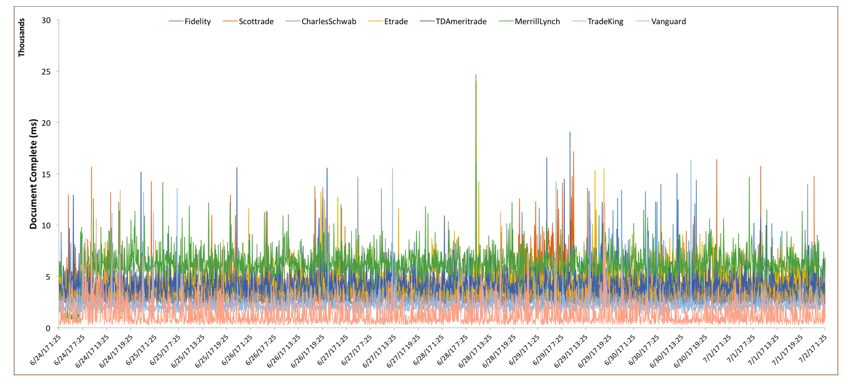Comparative visual analysis of the plot above is not feasible. One could potentially downsample , however, one would lose information in the process. In the wake of high-volume and high-velocity data, an algorithmic analysis is no longer nice to have.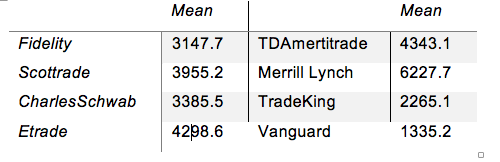The table above lists the mean value of Document Complete for each time series shown in the plot above. However, as it is well known, mean is susceptible to the presence of anomalies (which are indeed present in the plot above). Robust measures such as, but not limited to, trimmed mean, median or broadened median are commonly used. The latter, i.e., broadened median preserves the resistance of median with respect to anomalies while also achieving sensitivity to rounding and grouping of the values. The reader is referred to [1, 2] for further reading about robust measures.

The plot below shows the probability density distribution of the time series shown in the first plot.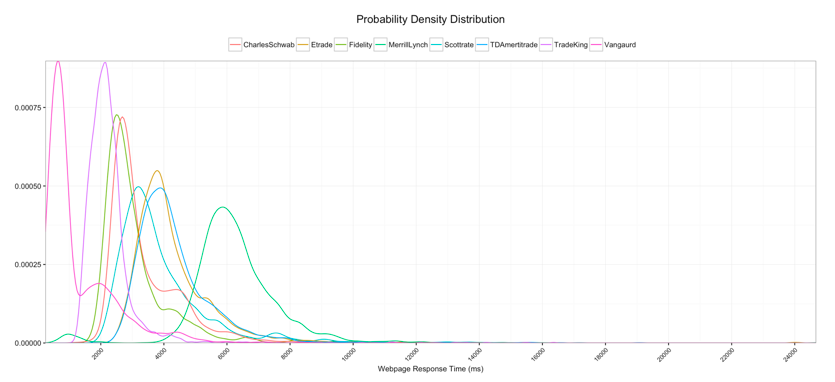From the plot above, we note that the distribution of none of the time series follows a normal distribution. This limits the use of certain quantile estimates, e.g., the method corresponding to argument type=8 in the R function quantile.

The table below lists the 50p, 95p and 99p estimates corresponding to argument type=7 in the R function quantile. From the table, we note that, for instance, although 50p and 95p of Etrade are much higher than that of Fidelity, 99p of Etrade is much higher that of Fidelity. Thus, percentiles do not necessarily follow a monotonic trend as exemplified by TDAmertitrade and TradeKing.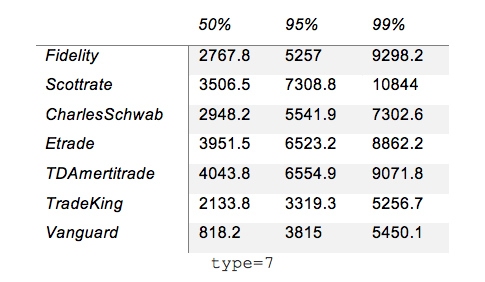The table below lists the 50p, 95p and 99p estimates corresponding to argument type=8 in the R function quantile. On comparative analysis of the tables above and below we note that the column corresponding to 50p is the same. However, the columns corresponding to 95p and 99p have different values. This has direct ramifications on multiple fronts. One of these corresponds to how SLA agreements put together (as discussed earlier here, breach of SLAs can have financial implications of the order of millions). Thus, it is important to be very specific about how quantile estimates should be computed.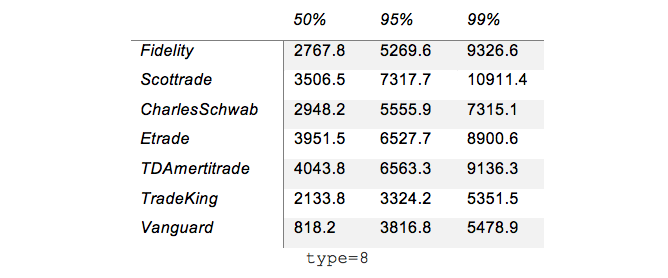We also note that the relative ordering of the brokerages does not change for 50p/95/99p between the two tables. However, from the table below we note that the ordering of 99.9p of Etrade relative to Scottrade and TDAmertitrade changed when transitioning from type=7 to type=8.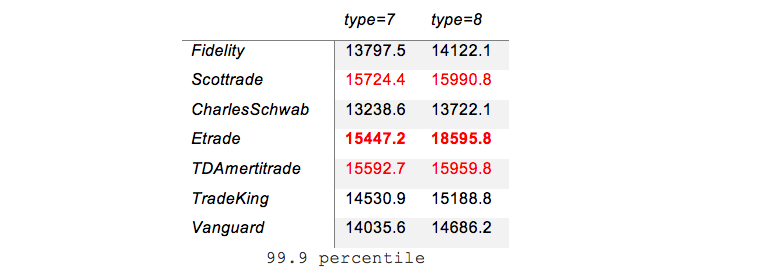This evidences the impact of the selection of a statistical method on comparative percentile analysis of ops data. This, in turn, can potentially have direct implications on investment of resources towards optimization.

A reader interested in learning more about quantile functions, he/she is referred to the evaluation paper by Schoojans et al.  and the book by Gilchrist . In the former, the authors stress the importance of reporting percentiles with their 95% confidence interval, esp. in the case of small samples.

To summarize, it is important to pay attention to the definition of percentile being used – be it when drafting contracts for SLAs or comparative performance analysis and optimization. Based on the literature, it is recommended to use the method corresponding to type=8 in R.

 “Understanding Robust and Exploratory Data Analysis,” by D. C. Hoaglin, F. Mosteller and J. W. Tukey.

 “Robust Statistics,” by P. J. Huber and Elvezio M. Ronchetti.

 “Sample quantiles in statistical packages,” by R. J. Hyndman and Y. Fan. In American Statistician 50, 361–365, 1996.

 “Sampling techniques,” W. G. Cochran.

 “Estimation of population percentiles,” by F. Schoonjans, D. De Bacquer and P. Schmid. In Epidemiology, 22(5): 750–751, 2011.

 “Statistical Modelling with Quantile Functions,” by W. Gilchrist.

By: Arun Kejariwal, Mehdi Daoudi, and Drit Suljoti

Web Experience
Application Availability
SLA Management
Media and Entertainment

Blog post

Blog post

Blog post

Blog post